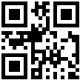author Sigbjorn FinneThu, 5 Mar 2009 02:24:10 +0000 (18:24 -0800) committer Sigbjorn FinneThu, 5 Mar 2009 02:24:10 +0000 (18:24 -0800)

index 6f79d71..ef0c0bf 100644 (file)
@@ -16,17 +16,24 @@ module Codec.Binary.UTF8.String (
, decode
, encodeString
, decodeString
+
+    , isUTF8Encoded
+    , utf8Encode
) where

-import Data.Word        (Word8)
+import Data.Word        (Word8,Word32)
import Data.Bits        ((.|.),(.&.),shiftL,shiftR)
import Data.Char        (chr,ord)

default(Int)

+-- | Encode a string using 'encode' and store the result in a 'String'.
encodeString :: String -> String
encodeString xs = map (toEnum . fromEnum) (encode xs)

+-- | Decode a string using 'decode' using a 'String' as input.
+-- | This is not safe but it is necessary if UTF-8 encoded text
+-- | has been loaded into a 'String' prior to being decoded.
decodeString :: String -> String
decodeString xs = decode (map (toEnum . fromEnum) xs)

@@ -62,13 +69,20 @@ decode [    ] = ""
decode (c:cs)
| c < 0x80  = chr (fromEnum c) : decode cs
| c < 0xc0  = replacement_character : decode cs
-  | c < 0xe0  = multi_byte 1 0x1f 0x80
+  | c < 0xe0  = multi1
| c < 0xf0  = multi_byte 2 0xf  0x800
| c < 0xf8  = multi_byte 3 0x7  0x10000
| c < 0xfc  = multi_byte 4 0x3  0x200000
| c < 0xfe  = multi_byte 5 0x1  0x4000000
| otherwise = replacement_character : decode cs
where
+    multi1 = case cs of
+      c1 : ds | c1 .&. 0xc0 == 0x80 ->
+        let d = ((fromEnum c .&. 0x1f) `shiftL` 6) .|.  fromEnum (c1 .&. 0x3f)
+        in if d >= 0x000080 then toEnum d : decode ds
+                            else replacement_character : decode ds
+      _ -> replacement_character : decode cs
+
multi_byte :: Int -> Word8 -> Int -> [Char]
where
@@ -84,3 +98,60 @@ decode (c:cs)

aux _ rs     _ = replacement_character : decode rs

+
+-- | @utf8Encode str@ is a convenience function; checks to see if
+-- @str@ isn't UTF-8 encoded before doing so. Sometimes useful, but
+-- you are better off keeping track of the encoding so as to avoid
+-- the cost of checking.
+utf8Encode :: String -> String
+utf8Encode str
+ | isUTF8Encoded str = str
+ | otherwise         = encodeString str
+
+
+-- | @isUTF8Encoded str@ tries to recognize input string as being in UTF-8 form.
+isUTF8Encoded :: String -> Bool
+isUTF8Encoded [] = True
+isUTF8Encoded (x:xs) =
+  case ox of
+    _ | ox < 0x80  -> isUTF8Encoded xs
+      | ox > 0xff  -> False
+      | ox < 0xc0  -> False
+      | ox < 0xe0  -> check1
+      | ox < 0xf0  -> check_byte 2 0xf 0
+      | ox < 0xf8  -> check_byte 3 0x7  0x10000
+      | ox < 0xfc  -> check_byte 4 0x3  0x200000
+      | ox < 0xfe  -> check_byte 5 0x1  0x4000000
+      | otherwise  -> False
+ where
+   ox = toW32 x
+
+   toW32 :: Char -> Word32
+   toW32 ch = fromIntegral (fromEnum ch)
+
+   check1 =
+    case xs of
+     [] -> False
+     c1 : ds
+      | oc .&. 0xc0 /= 0x80 || d < 0x000080 -> False
+      | otherwise -> isUTF8Encoded ds
+      where
+       oc = toW32 c1
+       d = ((ox .&. 0x1f) `shiftL` 6) .|.  (oc .&. 0x3f)
+
+   check_byte :: Int -> Word32 -> Word32 -> Bool
+   check_byte i mask overlong = aux i xs (ox .&. mask)
+      where
+        aux 0 rs acc
+         | overlong <= acc &&
+          acc <= 0x10ffff &&
+           (acc < 0xd800 || 0xdfff < acc) &&
+           (acc < 0xfffe || 0xffff < acc) = isUTF8Encoded rs
+         | otherwise = False
+
+        aux n (r:rs) acc
+         | toW32 r .&. 0xc0 == 0x80 =
+           aux (n-1) rs  (acc `shiftL` 6 .|. (toW32 r .&. 0x3f))
+
+        aux _ _  _ = False
+